## Posts

### Computational number theory in Sidef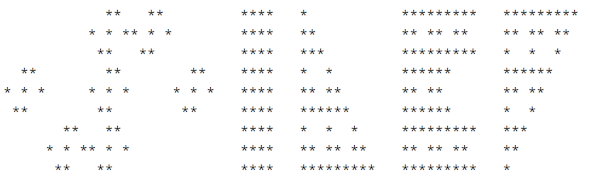In this tutorial we're going to look how we can use Sidef for doing various computations in number theory.

### Lossless data compression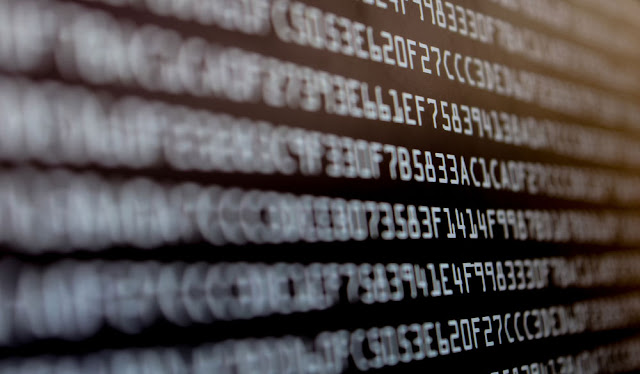Lossless data compression is the art of making files smaller, by applying various clever techniques designed to take advantage of the patterns in a file in order to create a compressed version that uses less space, but can be decompressed to form the original file.

### Digital steganography: hiding data in images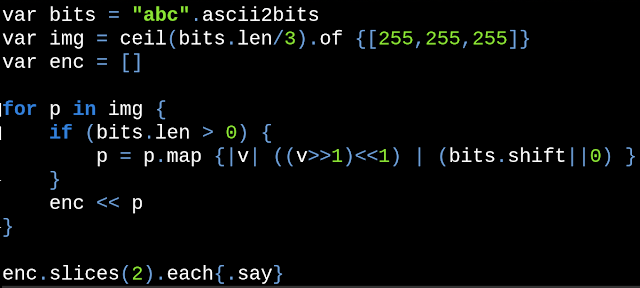Digital steganography is the practice of hiding that a communication is happening, by concealing a secret message into digital documents, without using a secret channel of communication, such that only someone that knows that a communication is happening and also knows the decoding method, is able to retrieve the hidden message.

### Pseudoprimes: construction methodsIn this post we're going to look at several methods for generating various kinds of  pseudoprimes and we're also listing some open-problems that motivate the generation of such numbers.

### Primality testing algorithmsIn this post we're going to look at some algorithms for checking if a given number is either prime or composite. Édouard Lucas ,  Leonhard Euler ,  Carl Pomerance  and  Pierre de Fermat Prime numbers play an important role in public-key cryptography (e.g. RSA algorithm ) which require fast generation of large random prime numbers that have around 600 digits (or more). The simplest way to generate a prime number, is to pick a random odd integer and check its primality, repeating this process until a prime is found. This approach requires a fast algorithm for checking the primality of a number. In 2002, Manindra Agrawal , Neeraj Kayal , and Nitin Saxena published the AKS primality test , unconditionally proving that the primality of a number can be checked in polynomial time, with an asymptotic time complexity of Õ(log(n)^12). Later on, in 2005, Carl Pomerance and Hendrik Lenstra improved the complexity of the AKS algorithm to run in just Õ(log(n)^6) steps.

### Primorial in algorithmsIn this post we present several practical algorithms based on  primorials .

### Special-purpose factorization algorithmsIn this post, we will take a brief look at a nice collection of special-purpose factorization algorithms. Most of them old and well-known. Some of them new.

### Partial sums of arithmetical functionsIn this post we're going to look at some very interesting generalized formulas for computing the partial sums of some arithmetical functions .

### Continued fraction factorization methodThe factorization method that we'll discuss in this post, it's called the  continued fraction factorization method  (CFRAC), and is quite an old method, but still pretty interesting, sharing many concepts and ideas with other modern factorization methods.

### Curiosities in number theoryIn this post I would like to present some interesting exercises in number theory , along with some curious formulas and identities for some number-theoretic functions .

### Investigating the Fibonacci numbers modulo m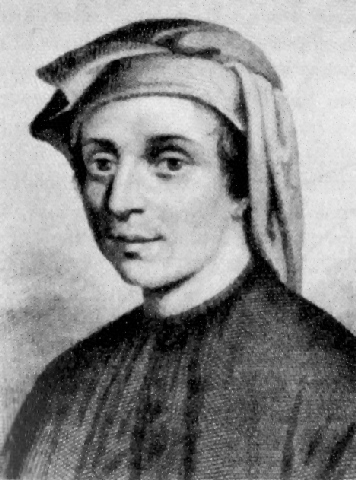The  Fibonacci sequence  is, without doubt, one of the most popular sequences in mathematics and in popular culture, named after Italian mathematician Leonardo of Pisa (also known as  Fibonacci , Leonardo Bonacci, Leonardo of Pisa, Leonardo Pisano Bigollo, or Leonardo Fibonacci), who first introduced the numbers in Western European with his book  Liber Abaci , in 1202.

### Representing integers as the sum of two squares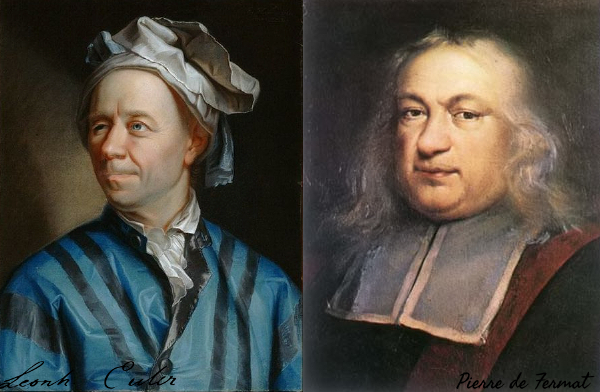In this post we present a recursive algorithm for finding all the possible representations, as a sum of two squares, for any given integer that can be expressed this way.

### Representing integers as the difference of two squaresMost integers can be represented as a difference of two squares, where each square is a non-negative integer.

### Various representations for famous mathematical constants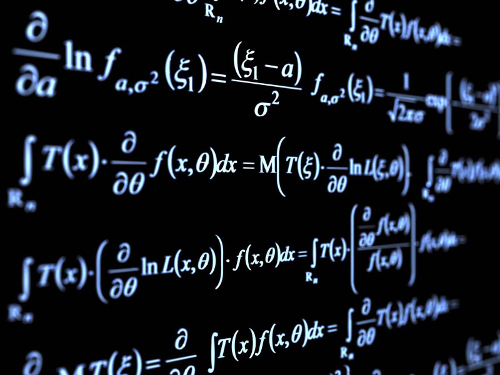In this unusual post, much like in the older post,  The beauty of Infinity , we're listing the most famous mathematical constants as representations of  infinite series ,  infinite products  and limits .

### Thoughts on programming language notationsSome posts ago, we looked at what it's required in creating a new programming language . In this post we're going a little bit more into it, trying to find ways to effectively express meanings in natural ways, similar to what we can express in a natural language.

### Bacovia: a symbolic math library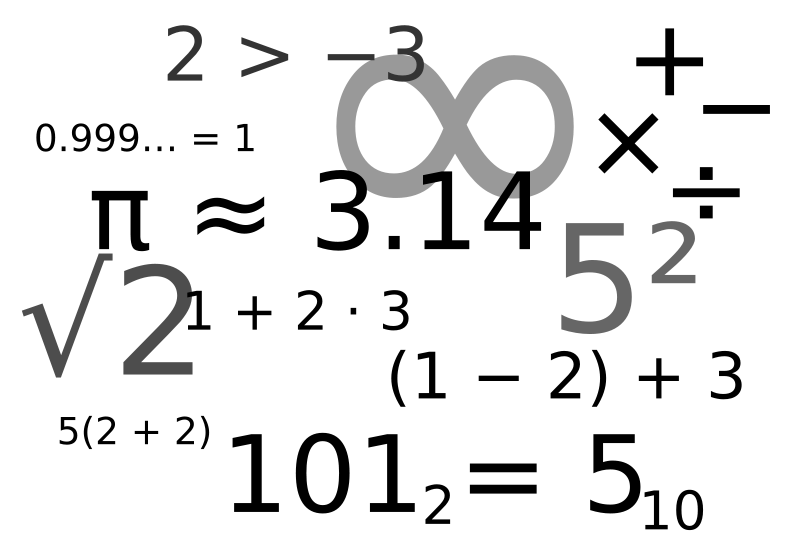Named after the great symbolist poet, George Bacovia , I created this library to symbolically manipulate mathematical expressions in a simple and elegant way.

### Mandelbrot setThe Mandelbrot set and its complex beauty.

### RSA algorithm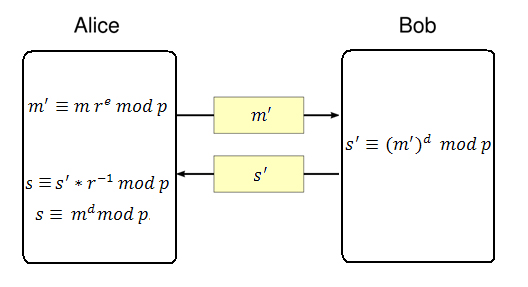RSA is a practical public-key cryptographic algorithm, which is widely used on modern computers to communicate securely over large distances. The acronym of the algorithm stands for Ron Rivest , Adi Shamir and Leonard Adleman , which first published the algorithm in 1978. # Algorithm overview Choose p and q as distinct prime numbers Compute n as n = p*q Compute \phi(n) as \phi(n) = (p-1) * (q-1) Choose e such that 1 < e < \phi(n) and e and \phi(n) are coprime Compute the value of d as d ≡ e^(-1) mod \phi(n) Public key is (e, n) Private key is (d, n) The encryption of m as c, is c ≡ m^e mod n The decryption of c as m, is m ≡ c^d mod n # Generating p and q In order to generate a public and a private key, the algorithm requires two distinct prime numbers p and q, which are randomly chosen and should have, roughly, the same number of bits. By today standards, it is recommended that each prime number to have a